# RF,GBDT and XGboost

Posted by GwanSiu on October 29, 2017

## 1. What‘s the emsembling learning?## 2. The basic concept of bootstrap, bagging and boost.

Bootstrap: bootstrap是一种有放回的抽样方法，在机器学习中又称自助法。Bootstrap在进行小样本估计时，效果更好。通过对子样本统计量的方差估计，进而构造置信区间。其思想和步骤如下：

1. 使用有放回的采样技术，从原有的样本集合中抽取一定数量的样本作为子样本集合。
2. 计算子样本集合的统计量T。
3. 重复(1)(2)步骤N次，得到N个不同的统计量T。
4. 计算N个统计量T的方差，得到统计量的方差，进而构造想相对应的置信区间。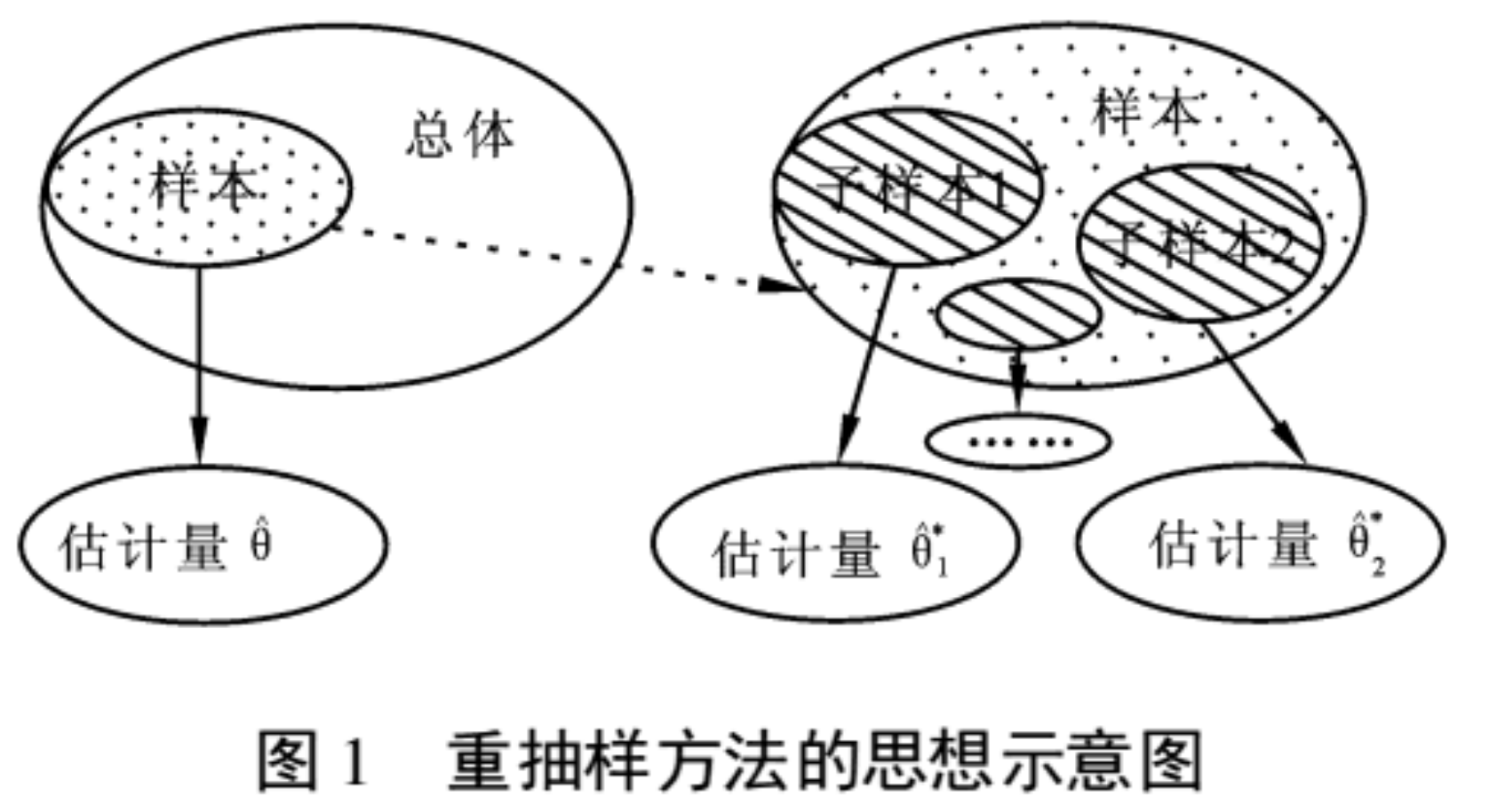Bagging(Boostrap aggregation): 从训练集中使用Bootstrap的方法采样(有放回采样)样本子集合，分别在这些子集合上训练分类器，得到一组函数序列$f_{1},…,f_{n}$, 最后将所有训练好的弱分类器进行组合形成强的分类器：对于分类问题则进行投票，对于回归问题则可以进行简单的平均的方法。Bagging的代表算法是Random Forest。Bagging的主要思想如下图：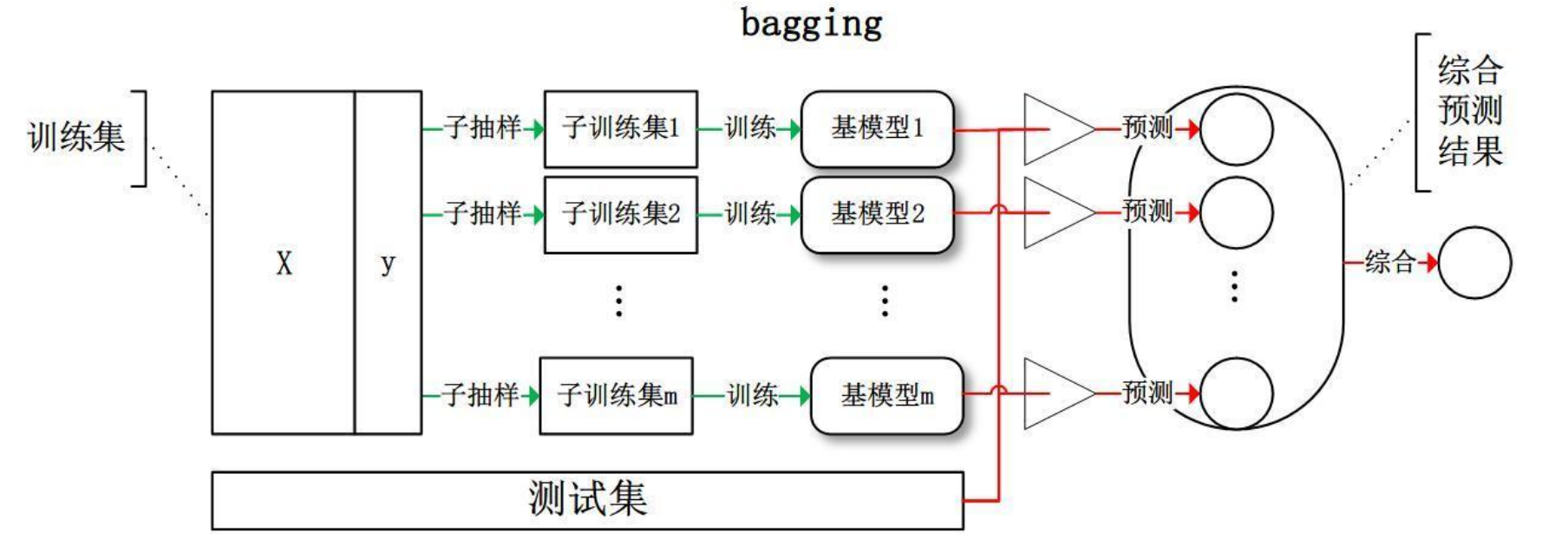Boosting:Boost同样是构造出一个函数序列(弱分类器)$f_{1},…,f_{n}$,与bagging不同的是，在boost算法框架下，后一个分类器依靠前一个分类器来生成：$f_{n}=F(f_{n-1})$。Boost算法更关注前一次错分的样本，boost算法的代表作是Adaboost，GDBT,xgboost算法。

## 3.Summary about bagging and boosting.

Bagging和boosting的区别主要在四个方面:

1. 采样方式：Bagging采用的是均匀采样的方式，而boosting则是根据错误率来进行采样，因此，boosting算法的精度要优于Bagging.
2. 训练集的选择: Bagging是随机选择训练集，在迭代中，训练集之间是相互独立的，而Boosting的训练集的选择则与前一次学习的结果有关。
3. 弱分类器: Bagging的训练出的弱分类没有权重，而在Boosting中，弱分类器是有权重的。
4. 计算方式:Bagging的各个弱分类器可以并行生成，而boosting的弱分类器只能按顺序生成。因此，bagging可以通过并行训练节省大量的时间开销。

Bagging算法可以并行训练很多的具有差异性且相互独立的弱分类器，这样的做法可以有效地降低最终模型的方差(variance)，因为采用了很多弱分类器后，最后得到的强分类器$f$会有效地逼近真实的函数$h$。因此，对于Bagging算法的每个弱分类器而言，关键是要降低模型的偏差(bias)，所以要采用深度很深甚至不剪枝的决策树。

## 4.Random Forest

### 4.1 The Algorithm of Random Forest

Random Forest算法分成四个部分:(1).随机选择样本(bootstrap);(2).随机选择特征；(3).构建决策树；(4).随机森林投票The Algorithm of Random ForestRandom Forest的随机性在于:样本的随机性，特征的随机性。从矩阵的角度上理解，假设行代表样本，列代表特征。Random Forest的随机性就随机抽样几行，在抽取的样本上在随机抽取几列特征。

### 4.2 Pros and Cons of Random Forest.

Random Forest的优点(在此引用):

1. 不容易出现过拟合，因为选择训练样本的时候就不是全部样本。
2. 可以既可以处理属性为离散值的量，比如ID3算法来构造树，也可以处理属性为连续值的量，比如C4.5算法来构造树。
3. 对于高维数据集的处理能力令人兴奋，它可以处理成千上万的输入变量，并确定最重要的变量，因此被认为是一个不错的降维方法。此外，该模型能够输出变量的重要性程度，这是一个非常便利的功能。
4. 分类不平衡的情况时，随机森林能够提供平衡数据集误差的有效方法

Random Forest的缺点(在此引用):

1. 随机森林在解决回归问题时并没有像它在分类中表现的那么好，这是因为它并不能给出一个连续型的输出。当进行回归时，随机森林不能够作出超越训练集数据范围的预测，这可能导致在对某些还有特定噪声的数据进行建模时出现过度拟合。
2. 对于许多统计建模者来说，随机森林给人的感觉像是一个黑盒子——你几乎无法控制模型内部的运行，只能在不同的参数和随机种子之间进行尝试。

## 5. Gradient Boost Decision Tree(GDBT)

### 5.1 Boosting Tree

\begin{aligned} f_{0}(x) &= 0 \\ f_{m}(x) &= f_{m-1}(x)+T(x;\Theta_{m}), \text(m=1,2,...,M) \\ f_{M}(x) &= \sum_{m=1}^{M}T(x;\Theta_{m}) \end{aligned}

Boost Tree的算法如下(引用李航统计学习):### 5.2 Gradient Boosting Decision Tree(GDBT)

Gradient Boosting方法是是梯度下降(Gradient descent)的想法应用在提升方法(Boosting)中，因为前向分步算法是一个残差逼近的过程，新学习的函数$\Theta_{m}$需要让残差进一步下降。Freid便提出梯度提升(Gradient Boosting)算法框架, 使用损失函数的负梯度作为残差的近似值(why？下面addicative model有解释)。具体的算法如下：### 5.2 Gradient Boosting Decision Tree(GDBT)

1. A loss function to be optimized.
2. A week learner to make predictions.
3. An addictive model to add weak learners to minimize the loss function.

1. 损失函数: 从GDBT算法中可以看出，GDBT算法要求损失函数必须是一阶可导的，而且GDBT框架下，任何一阶可导的损失函数都可以使用，不必再为其推导一个新的boosting算法。

2. 弱分类器: GDBT框架下使用的是决策树作为基函数，每一次迭代过程中，当前函数的构建是在前一次学习结果的基础上使用贪心算法，即：基于当前情况(Gini系数或者纯度)，选择最佳分裂点. 在学习弱分类器的过程中，可以对弱分类器加入约束，如:决策树可以约束深度和叶子的数量。

Traditionally, gradient descent is used to minimize a set of parameters, such as the coefficients in a regression equation or weights in a neural network. After calculating error or loss, the weights are updated to minimize that error.

Instead of parameters, we have weak learner sub-models or more specifically decision trees. After calculating the loss, to perform the gradient descent procedure, we must add a tree to the model that reduces the loss (i.e. follow the gradient). We do this by parameterizing the tree, then modify the parameters of the tree and move in the right direction by (reducing the residual loss.

Generally this approach is called functional gradient descent or gradient descent with functions

## 6. XGboost 模型

XGboost模型是基于GDBT模型的改进提高版，其算法如下:

img src=”http://static.zybuluo.com/GwanSiu/3pldqaxs88fsz3uri5oniq6h/image.png” width = “600” height = “300” alt=”abc”/>

XGboost使用的是CART树，对于GBDT提出四方面的修改:

1.利用函数二阶导来代替GDBT中的一阶导，使算法更快收敛。

\begin{aligned} L(\Phi) &=\sum_{i}(\hat{y_{i}},y_{i}) + \sum_{k}\Omega(f_{k}) \\ \Omega(f) &= y\gamma+\frac{1}{2}\lambda\Vert\omega\Vert^{2} \\ \end{aligned} 转化成: \begin{aligned} L^{t} &= \sum_{i=1}^{n} l(y_{i},\hat{y}_{i-1}^{t}+f_{t}(x_{i}))+\Omega(f_{t})\\ L^{t} &\approx \sum_{i=1}^{n}[l(y_{i}, \hat{y}^{(t-1)})+g_{i}f_{t}(x_{i})+\frac{1}{2}h_{i}f^{2}_{t}(x_{i})]+\Omega(f_{t}) \\ \text{where } g_{i} &= \partial_{\hat{y}^{(t-1)}}l(y_{i},\hat{y}^{(t-1)})\\ h_{i} &= \partial_{\hat{y}^{(t-1)}}^{2}l(y_{i},\hat{y}^{(t-1)})\\ \Rightarrow L^{t} &\approx \sum_{i=1}^{n}[g_{i}f_{t}(x_{i})+\frac{1}{2}h_{i}f^{2}_{t}(x_{i})]+\Omega(f_{t}) \\ \end{aligned}

2.模型的约束(叶子节点，树的深度等)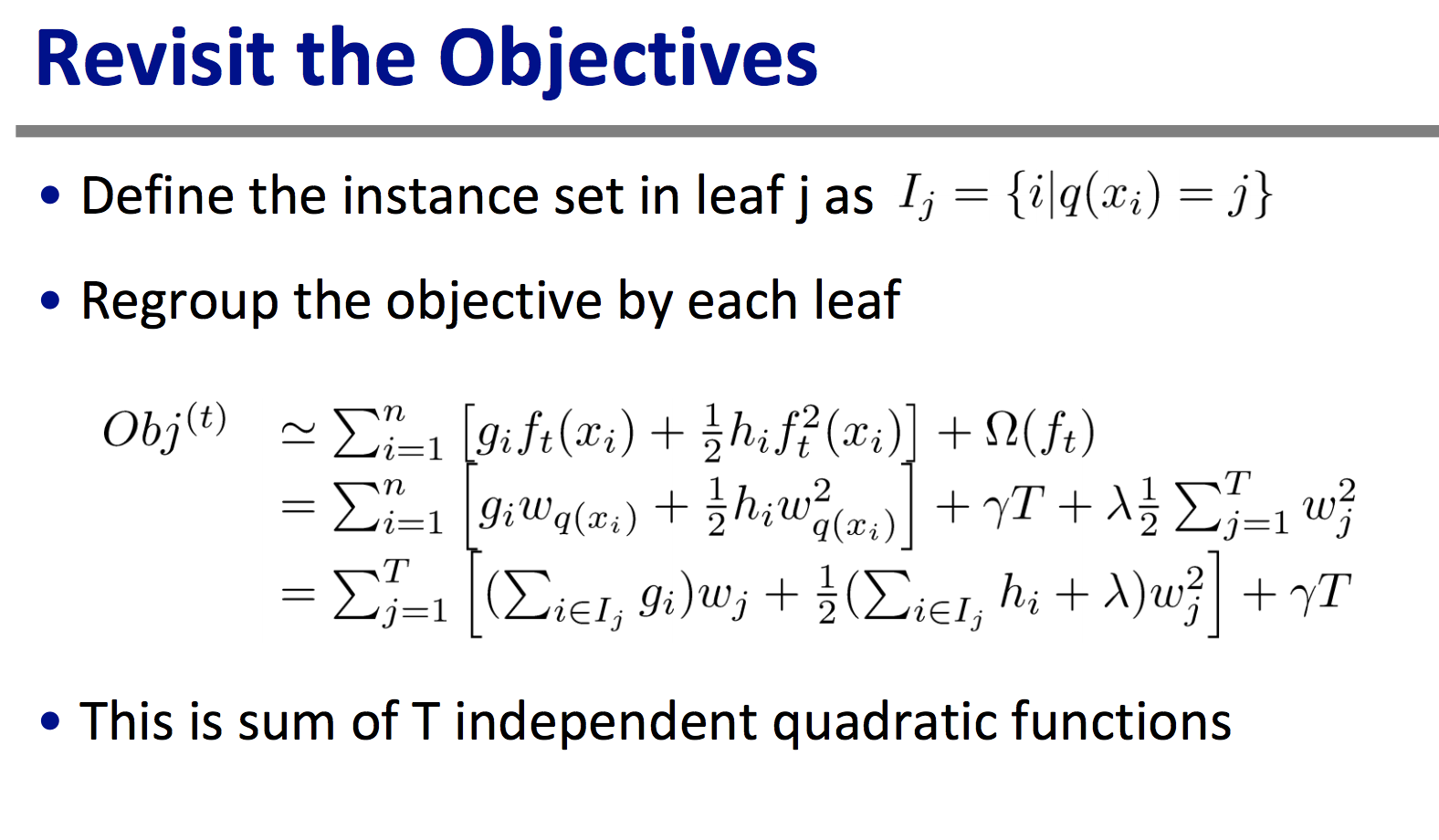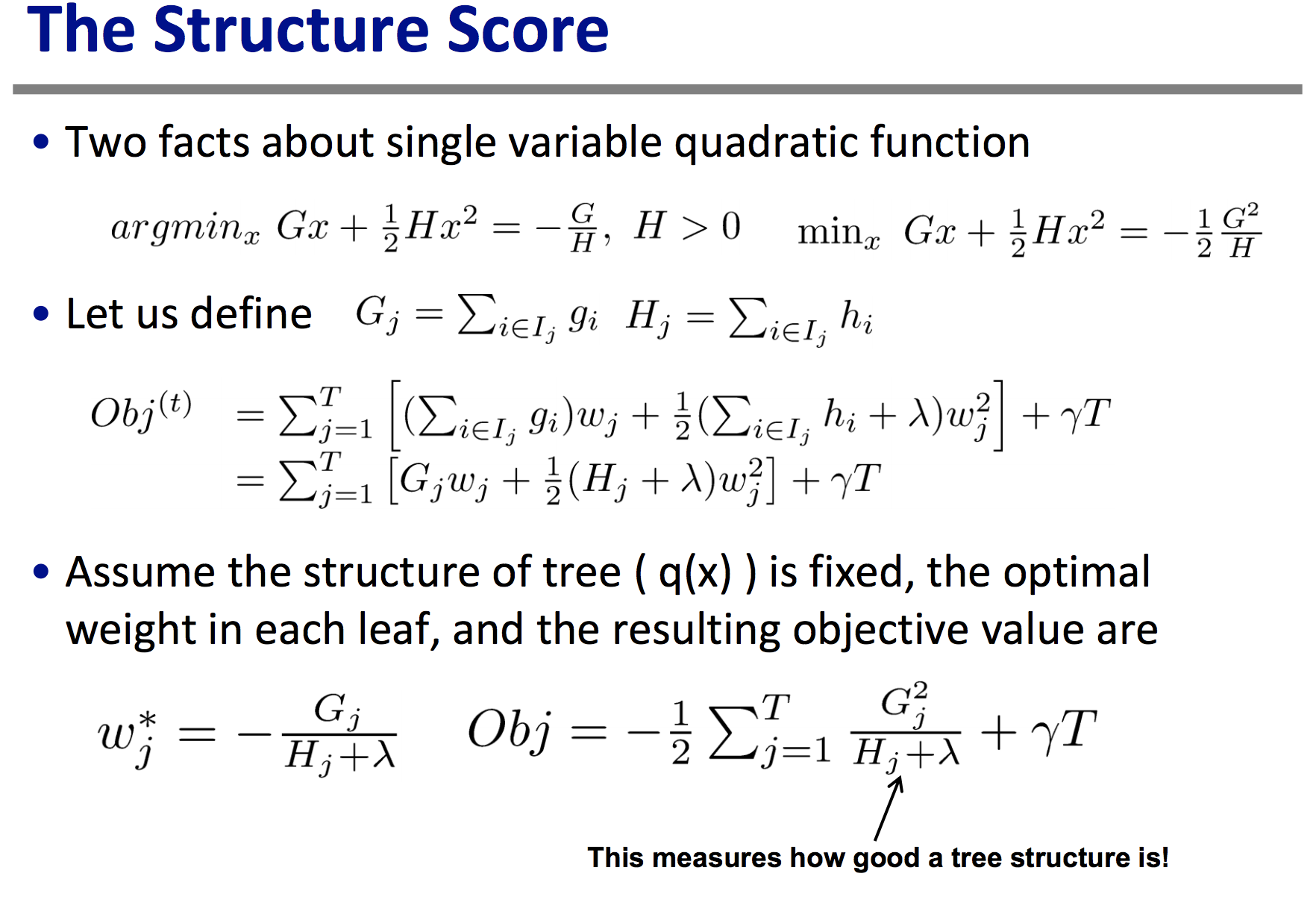3.自动寻出最佳裂项节点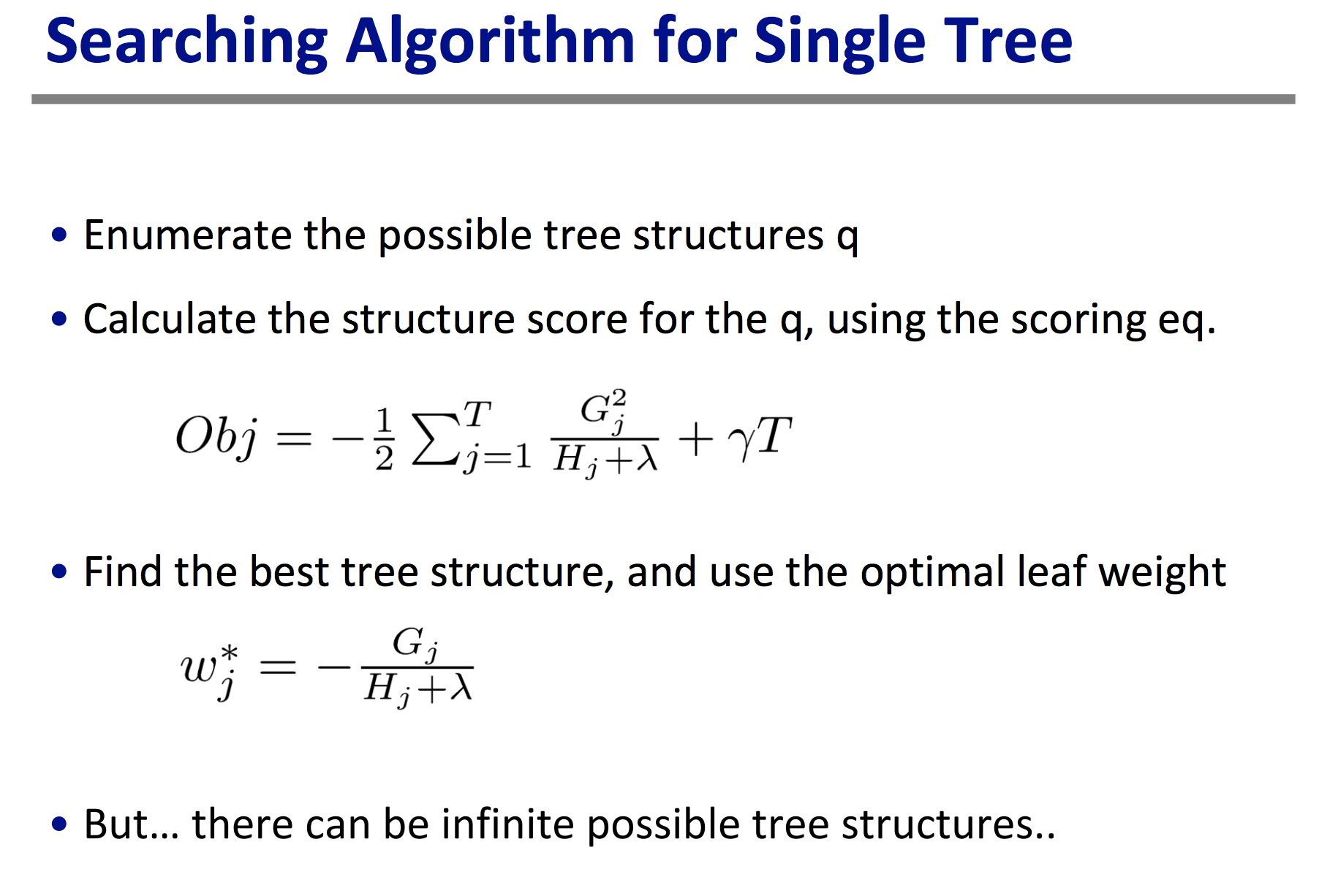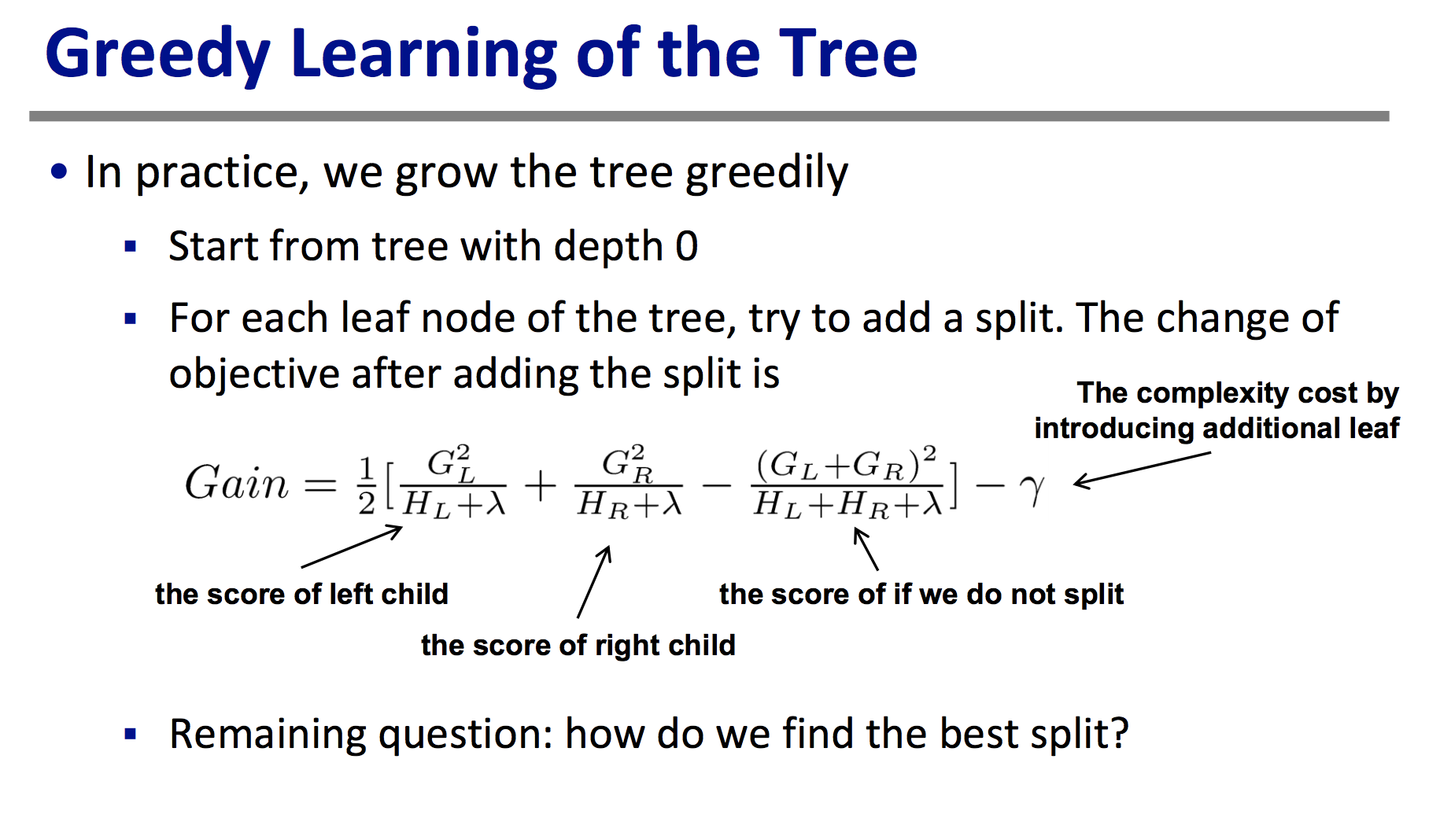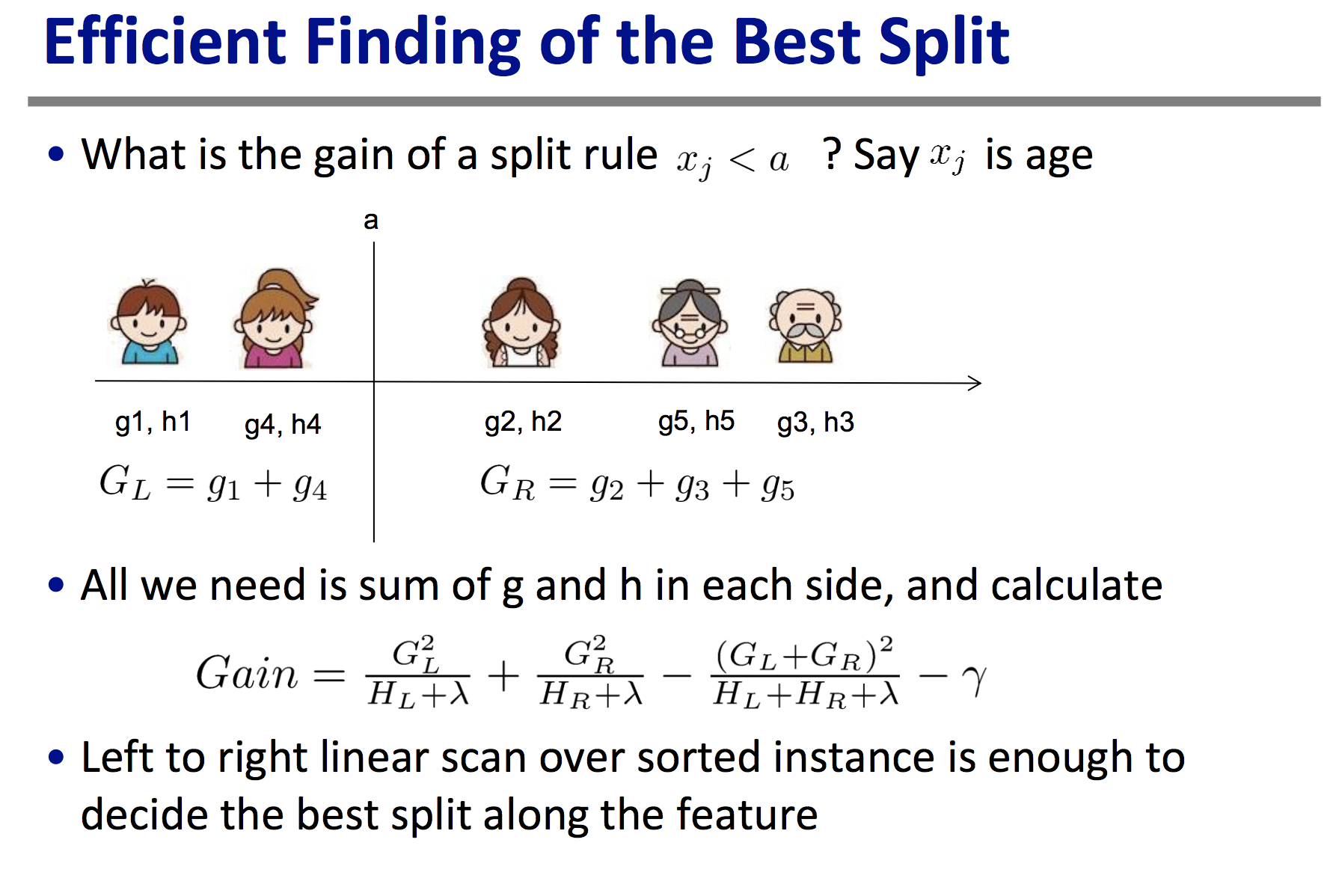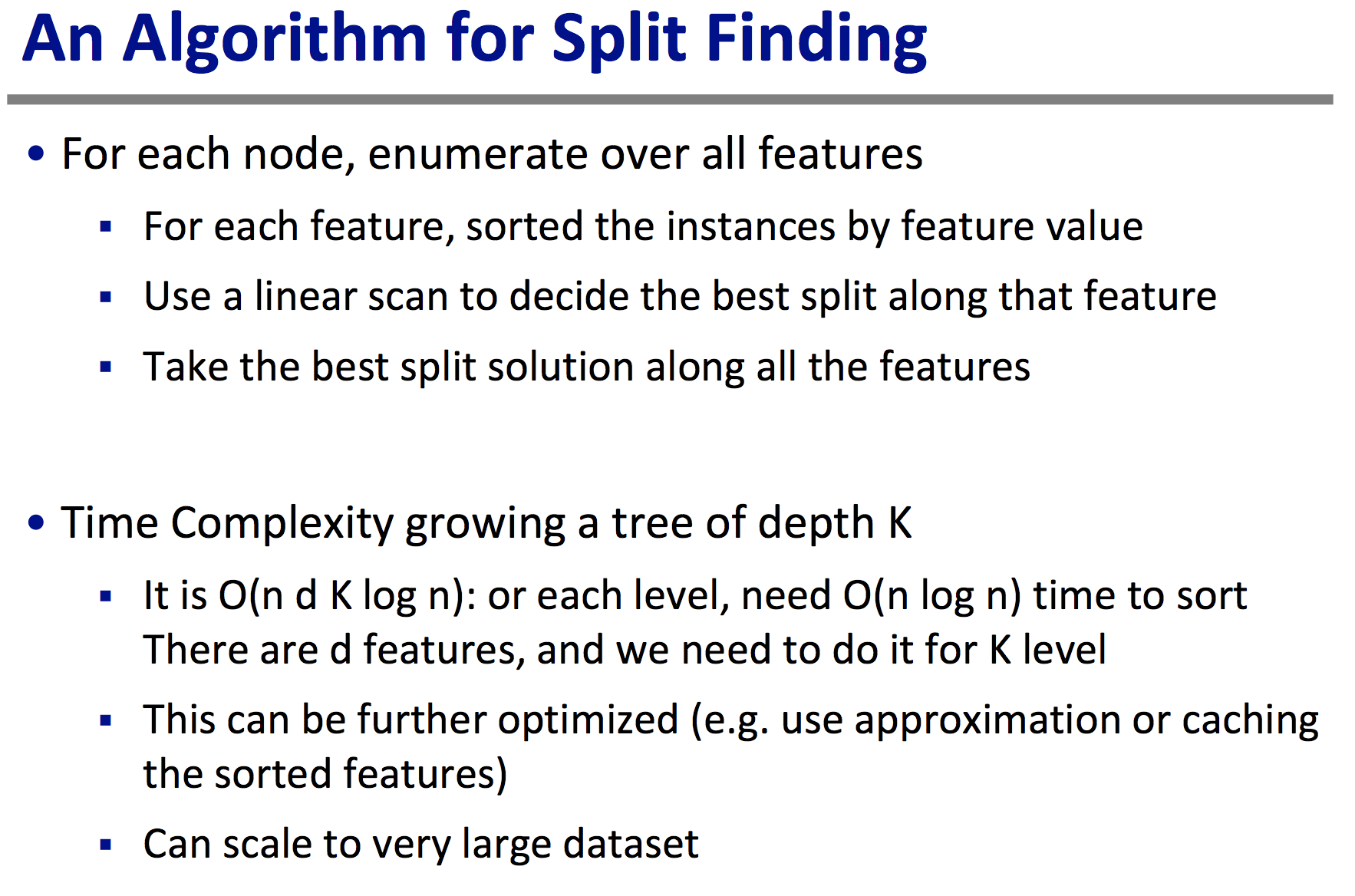4.类别变量的处理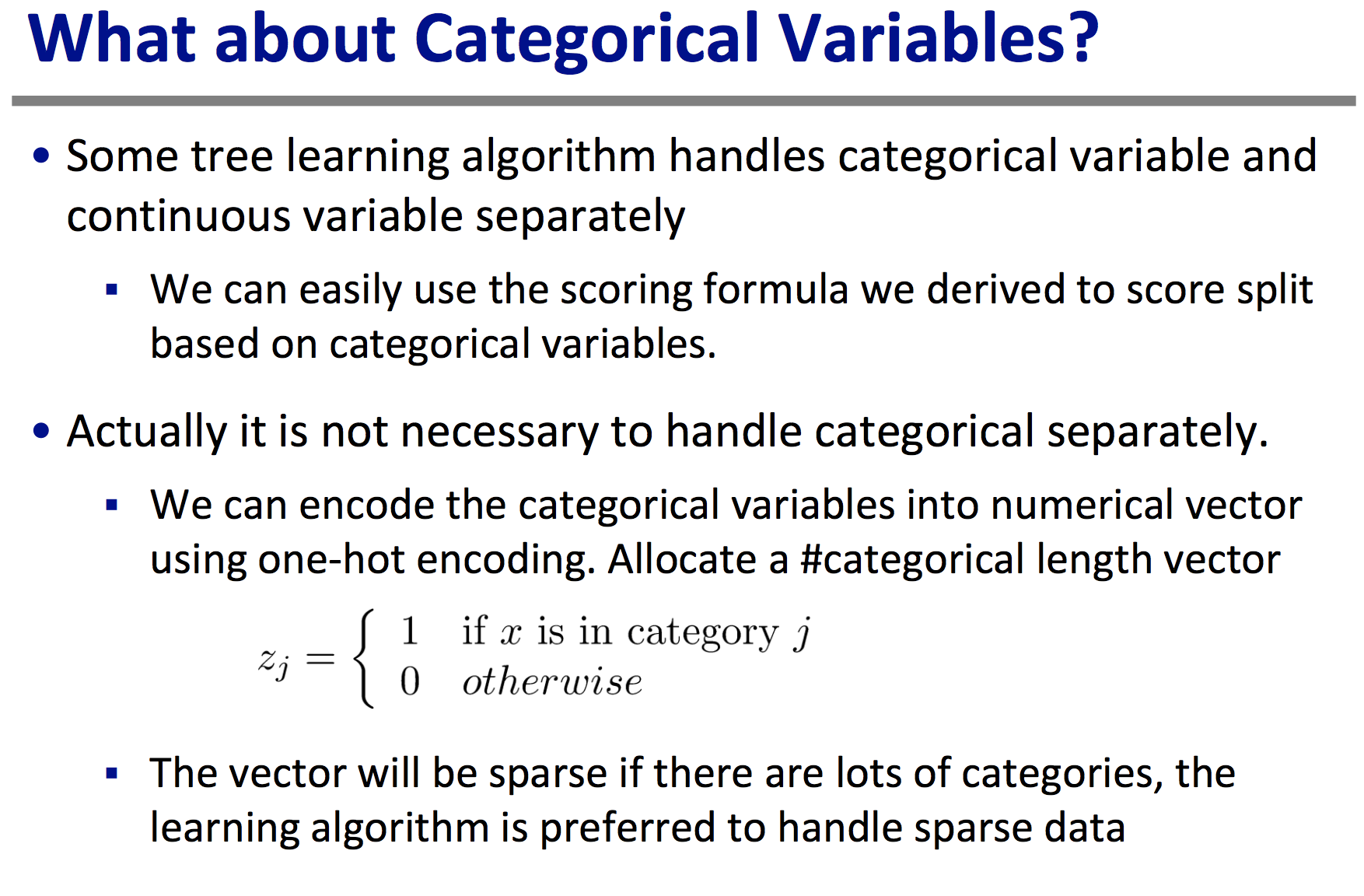5.Regulization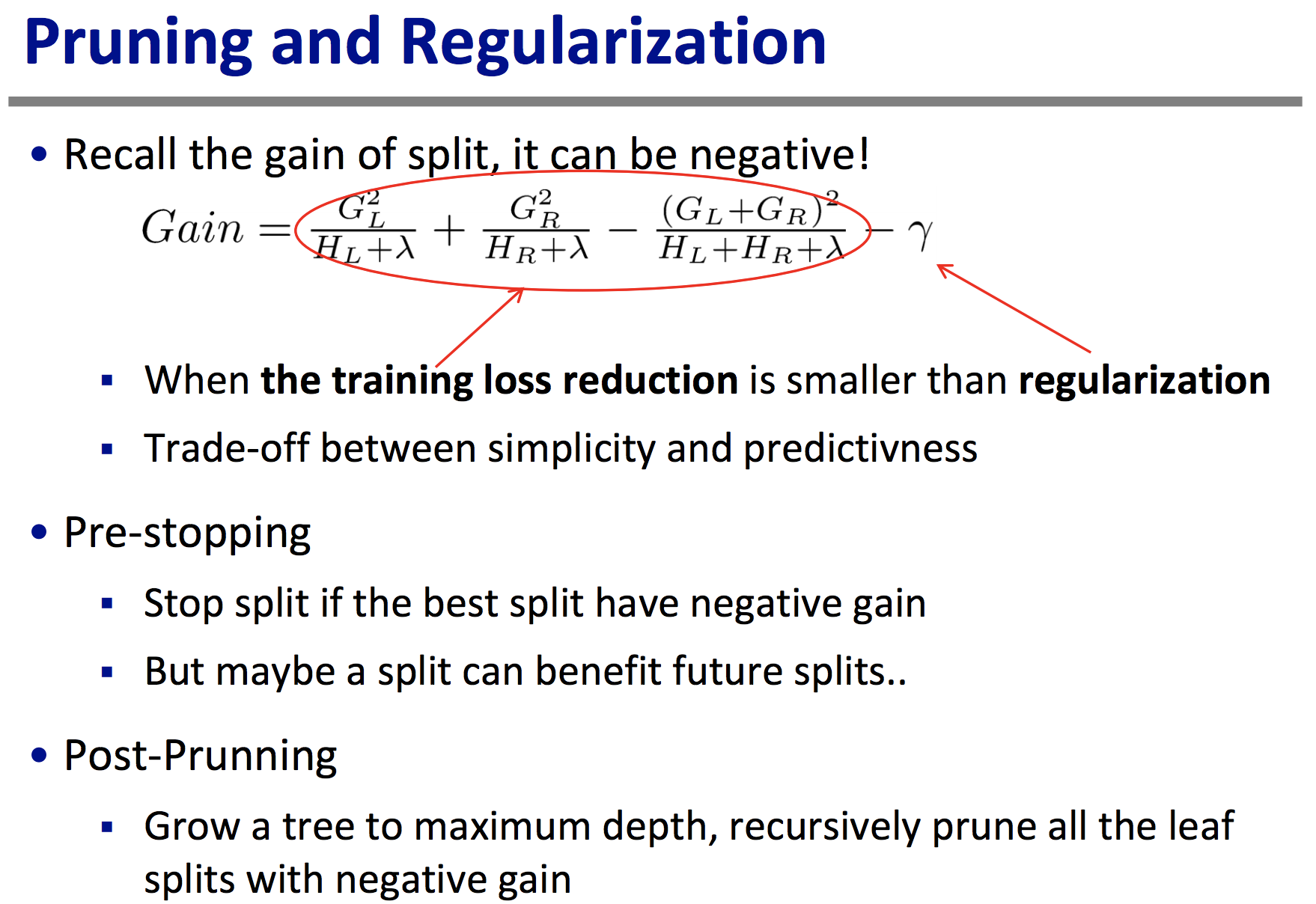http://blog.sina.com.cn/s/blog_4a0824490102vb2c.html(http://blog.sina.com.cn/s/blog_4a0824490102vb2c.html)
 http://www.jianshu.com/p/005a4e6ac775(http://www.jianshu.com/p/005a4e6ac775)
 统计学习方法，李航.  https://homes.cs.washington.edu/~tqchen/pdf/BoostedTree.pdf